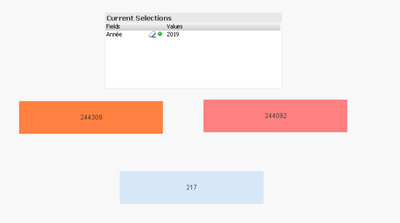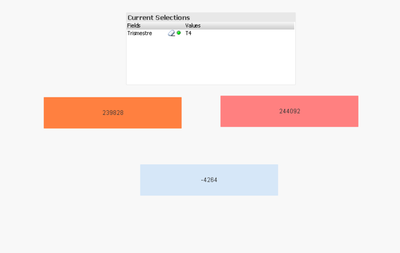# QlikView Integrations

Discussion Board for collaboration on QlikView Integration.

Announcements
Our next Qlik Insider session will cover new key capabilities. Join us August 11th REGISTER TODAY
cancel
Showing results for
Did you mean:Contributor III

## Calculate the delta between two end dates

Hi everyone !

I am contacting you to request your assistance.

I would like to create a line chart
which calculates delta between  N and N-1 (years, semester, quarter, month, days).
I made an hierarchy for that.

The blocking point is that the calculation must always select the last date of the selected period.

For example:

If I'm on a view per month,
I would calculate 31/01/2010 - 31/01/2019 and the same for all months.

Thanks a lot

Labels (3)

• ### Last date

1 Solution

Accepted SolutionsMaster II

with the attached version, I think we have the same values.The alternative is to calculate it in script and then apply it in measure

S:

``````=if(isnull(Max({\$<YEAR={\$(=Max(YEAR))}>} S_CONTRACT_COUNT)),0,Max({\$<YEAR={\$(=Max(YEAR))}>} S_CONTRACT_COUNT))
-if(isnull(Max({\$<YEAR={\$(=Max(YEAR)-1)}>} S_CONTRACT_COUNT)),0,Max({\$<YEAR={\$(=Max(YEAR)-1)}>} S_CONTRACT_COUNT))``````

T:

``````=if(isnull(Max({\$<YEAR={\$(=Max(YEAR))}>} T_CONTRACT_COUNT)),0,Max({\$<YEAR={\$(=Max(YEAR))}>} T_CONTRACT_COUNT))
-if(isnull(Max({\$<YEAR={\$(=Max(YEAR)-1)}>} T_CONTRACT_COUNT)),0,Max({\$<YEAR={\$(=Max(YEAR)-1)}>} T_CONTRACT_COUNT))``````

Regards,
Taoufiq ZARRA

"Please LIKE posts and "Accept as Solution" if the provided solution is helpful "

(you can mark up to 3 "solutions") 😉
25 RepliesMaster II

can you share a sample data and the expected output

Regards,
Taoufiq ZARRA

"Please LIKE posts and "Accept as Solution" if the provided solution is helpful "

(you can mark up to 3 "solutions") 😉Contributor III
Author

Hi @Taoufiq_Zarra .

Here is an example of data.

There are dates informations, an other dimension CITY, and a measure NB.

I would like to make a line chart visualization  which calculate delta between Year N and Year N-1 for all date element (delta = Measure N - Measure N-1)
I have to do calculation with last dat eof each periode.

In the picture, there is a filter (green) named "Dimensions".
I have to be able to display my measure by fo example CITY (or another dim).

Thanks a lot

Best Regards,

HarryMaster II

Bonjour @marakud971

You can add for example {\$<Year={"\$(=Max(Year))"}>} for Year and {\$<Year={"\$(=Max(Year)-1)"}>} for YEAR-1

I can't suggest the exact script since I haven't understood what you want to do yet.

Can you give more detail or ana example.

Merci pour ton retour

Regards,
Taoufiq ZARRA

"Please LIKE posts and "Accept as Solution" if the provided solution is helpful "

(you can mark up to 3 "solutions") 😉Contributor III
Author

sorry, i will give you more explanations.

For example :

if in my visualization, i've got a hierachy Year-> Semester->Quarter-Month->Week->Day

• if i'm in the year level, i would like do to : max(2020) - max(2029)
• if i'm in the semester level, i would like do to max(Semester 1 of 2020) - max(Semester 1 of 2019) and max(Semester 2 of 2020) - max(Semester 2 of 2019). In my line chart semesters are on axis X.
• if i'm in the quarter level, i would like do to max(quarter 1 of 2020) - max(quarter 1 of 2019) and max(quarter 2 of 2020) - max(quarter 2 of 2019) and max(quarter 3 of 2020) - max(quarter 3 of 2019)....etc...
• About Week it's the same logic.

I have to find the last date of each period when i calculate the difference.

In a second part,

i have to aggregate this measure if i would like to display a dim values in my chart.

Is my need clearer ?

Thanks @Taoufiq_ZarraMaster II

If I understand correctly, you always compare the max year (or the selected year) with the year -1.
so I think you just need :

``=Max({\$<Année={"\$(=Max(Année))"}>}  CONTRACT_COUNT)-Max({\$<Année={"\$(=Max(Année)-1)"}>}  CONTRACT_COUNT)``

in the other levels if you select, month, semester, ..., the same formula will work, since it will compare the selected month, semester,... of the year with year-1.attached qvw file

Don't hesitate if you have any other remarks.

Regards,
Taoufiq ZARRA

"Please LIKE posts and "Accept as Solution" if the provided solution is helpful "

(you can mark up to 3 "solutions") 😉Contributor III
Author

thanks @Taoufiq_Zarra .

I tried your solution. It works well in a textbox. Thanks !

But how to adapt this measure in a line chart ?
When i try, my visualisazion stay blank.

Thanks again.Master II

@marakud971  how you  conceived the line chart, what do you expect for the x-axis and y?

Regards,
Taoufiq ZARRA

"Please LIKE posts and "Accept as Solution" if the provided solution is helpful "

(you can mark up to 3 "solutions") 😉Contributor III
Author
• x-axis : my date hierarchy
• y-axis : values
• Legend : colunm CITY

I updated my data file.
you'll find in attachment
You'll also find a picture in this xls file.

Regards,
HarryMaster II

@marakud971 I'm sorry, but I still can't fully understand the need ?
are the values is Max year-(Max year-1)?
can you give me a simple example to understand:

I want to display the XXXX values for T1 for MArtinique as an example?

attached the version

Regards,
Taoufiq ZARRA

"Please LIKE posts and "Accept as Solution" if the provided solution is helpful "

(you can mark up to 3 "solutions") 😉Community Browser